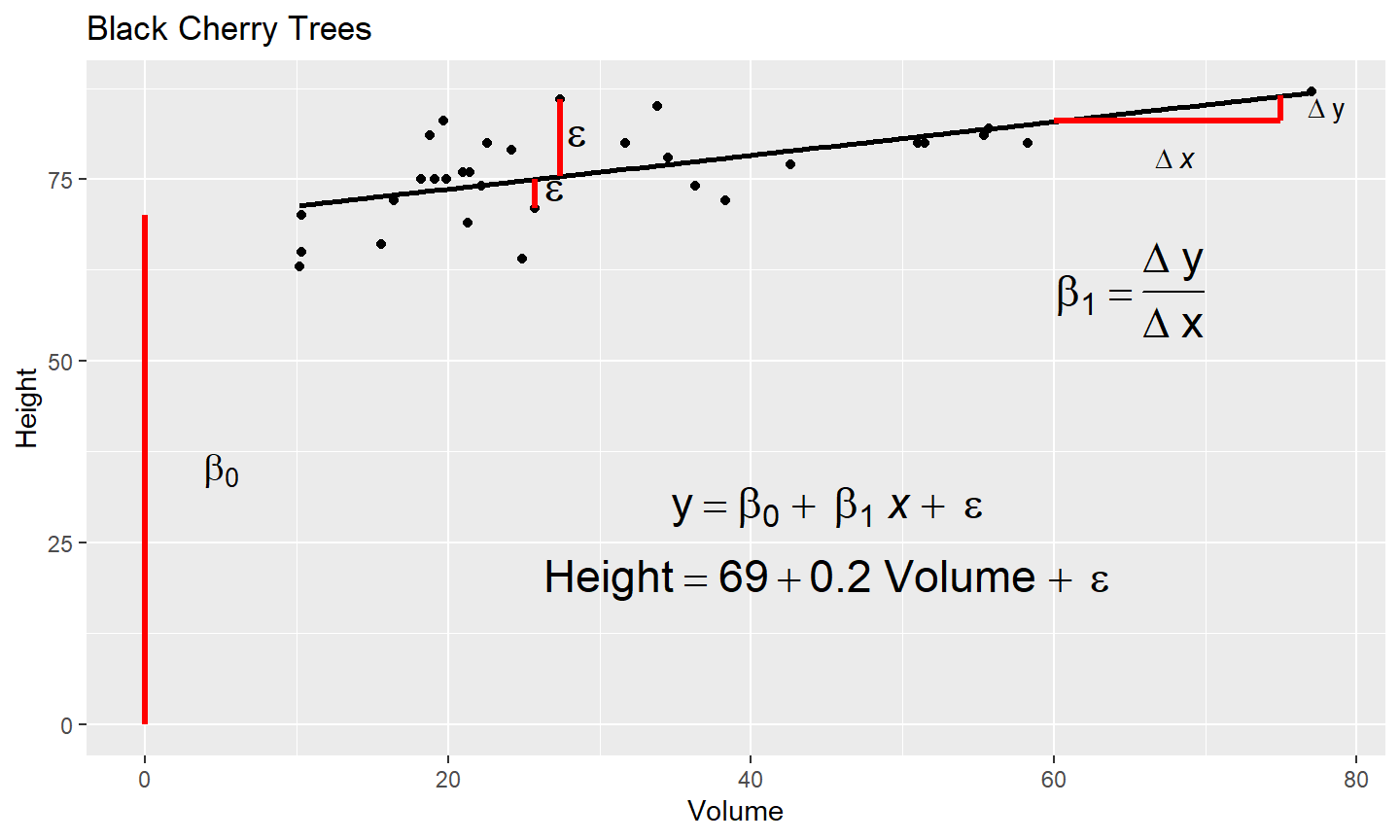May 12 2021

## Objectives

• Understand linear regression and describe a case study
• Compute and interpret coefficients in a linear regression analysis in R.
• Interpolate regression model in R to produce a raster layer.

## Science

What are we doing?

• Explain
• Predict

## Connections

Why is it windy in Iowa?

• Missouri sucks and Minnesota blows
• No connection

Where do babies come from?

• Storks deliver babies
• No connection

Why are basements in Iowa full of cracks?

• Soils in Iowa contain high amounts of shrink-swell clays
• Connection

## Predictions and Predicting with a Function

Predictions

• None of us will report to work on Sunday
• The average price of a gallon of gas in the US will be \$100.00 on January 1

Predicting with a function

• $y= \beta_0 + \beta_1x + \epsilon$

## Assumptions

• Linear
• Independent
• Homoscedastic
• Normal

## Interpreting the Linear Regression Model## Building the Linear Regression Model

• Ordinary Least Squares
• $\beta_1= \frac{\sum(x_i - \bar x) (y_i - \bar y)} {\sum(x_i - \bar x)^2}$
• $\beta_0= \bar y - \beta_1 \times \bar x$

## Examples

Wills et al., 2013

Carbon equivalent correction regression factor: $OC_{dc}= 0.25 + 0.86(OC_{wc})$ where

$$OC_{dc}=$$ organic carbon by dry combustion (%)

$$OC_{wc}=$$ organic carbon by wet combustion (%)